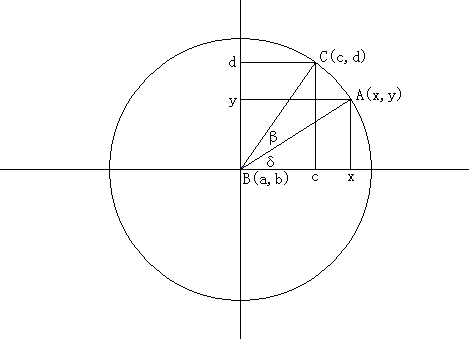• 6378.137 * 2 * asin(sqrt(pow(sin((lat1*pi()/180 - lat2*pi()/180)/2),2) + cos(lat1*pi()/180)*cos(lat2*pi()/180)*pow(sin( (lng1*pi()/180 - lng2*pi()/180)/2),2))) 2 * 6378.137 * asin(sqrt(pow(sin(pi
6378.137 * 2 * asin(sqrt(pow(sin((lat1*pi()/180 - lat2*pi()/180)/2),2) + cos(lat1*pi()/180)*cos(lat2*pi()/180)*pow(sin( (lng1*pi()/180
- lng2*pi()/180)/2),2)))2 * 6378.137 * asin(sqrt(pow(sin(pi()*(lat1 - lat2)/360),2) + cos(pi()* lat1 /180)*cos(lat2*pi()/180)*pow(sin(pi()*(lng1 - lng2)/360),2)))

au_around
==========================
id  ===  aname === blng === blat
==========================
$blat =$_GET["bdlat"];
$blng =$_GET["bdlng"];
$sql = "select *,(2*6378.137*ASIN(SQRT(POW(SIN(PI()*(".$blat." - blat)/360),2)+COS(PI()* ".$blat." /180)*COS(blat *PI()/180)*POW(SIN(PI()*(".$blng." - blng)/360),2)))) as juli from au_around order by juli asc limit 0, 20";

展开全文• 平面内直角坐标系中坐标旋转变换公式今天做数字图像处理作业时遇到一个关于图片旋转变换的问题，要用到坐标的旋转变换公式，突然不记得公式是怎么来的了，于是乎，就琢磨了一番。。。首先上公式：逆时针（如下图）：...
首先上公式：

逆时针（如下图）：
x1=xcos(β)-ysin(β);
y1=ycos(β)+xsin(β);

顺时针（图未给出）：
x1=xcos(β)+ysin(β);
y1=ycos(β)-xsin(β);

其中x，y表示物体相对于旋转点旋转β的角度之前的坐标，x1，y1表示物体旋转β后相对于旋转点的坐标。此公式仅为在下图坐标中的变换公式，坐标系的选取不同可能会有不同的结果，但是推导方式一样，请大家注意。

下面是推导过程：

从数学上来说，此公式可以用来计算某个点绕另外一点旋转一定角度后的坐标，例如：A（x，y）绕B（a，b)旋转β度后的位置为C（c，d），则x，y，a，b，β，c，d有如下关系式：1.设A点旋转前的角度为δ，则旋转(逆时针)到C点后角度为δ+β

2.求A，B两点的距离：dist1=|AB|=y/sin(δ)=x/cos(δ)

3.求C，B两点的距离：dist2=|CB|=d/sin(δ+β)=c/cos(δ+β)

4.显然dist1=dist2，设dist1=r所以：
r=x/cos(δ)=y/sin(δ)=d/sin(δ+β)=c/cos(δ+β)

5.由三角函数两角和差公式知：
sin(δ+β)=sin(δ)cos(β)+cos(δ)sin(β)
cos(δ+β)=cos(δ)cos(β)-sin(δ)sin(β)

所以得出：
c=r*cos(δ+β)=r*cos(δ)cos(β)-r*sin(δ)sin(β)=xcos(β)-ysin(β)
d=r*sin(δ+β)=r*sin(δ)cos(β)+r*cos(δ)sin(β)=ycos(β)+xsin(β)

即旋转后的坐标c，d只与旋转前的坐标x，y及旋转的角度β有关
从图中可以很容易理解出A点旋转后的C点总是在圆周上运动，圆周的半径为|AB|，利用这点就可以使物体绕圆周运动，即旋转物体。

另外，顺时针旋转可以理解为逆时针一个负角度，根据sin(),cos()的奇偶性，即sin(-β)=-sin(β),cos(-β)=cos(β),可得顺时针旋转的变换公式:

x1=xcos(β)+ysin(β);
y1=ycos(β)-xsin(β);
展开全文• 平面距离公式 准备知识 平面的一般式方程 Ax +By +Cz + D = 0 其中n = (A, B, C)是平面的法向量，D是将平面平移到坐标原点所需距离（所以D=0时，平面过原点） 向量的模（长度） 给定一个向量V（x, y, z)...
点到平面的距离公式

准备知识

平面的一般式方程

Ax +By +Cz + D = 0

其中n = (A, B, C)是平面的法向量，D是将平面平移到坐标原点所需距离（所以D=0时，平面过原点）

向量的模（长度）

给定一个向量V（x, y, z),则|V| = sqrt(x * x + y * y + z * z)

向量的点积（内积）

给定两个向量V1(x1, y1, z1)和V2(x2, y2, z2)则他们的内积是

V1V2 = x1x2 + y1y2 + z1z2

点到平面的距离

有了上面的准备知识，则求点到直线的距离不再是难事，有图有真相如果法相量是单位向量的话，那么分母为1

转载自：http://www.cnblogs.com/graphics/archive/2010/07/10/1774809.html

展开全文推导 公式
• 已知两点经纬度计算球面距离公式，一搜一大堆，形式如下： 可是至于这个公式为什么是这样的，今天推导了一下，详细推导过程如下。首先画个图（图1），要不然空间想象能力差的话容易犯糊涂。首先对图1做个大致的...
https://blog.csdn.net/yu412346928/article/details/42966001

已知两点经纬度计算球面距离的公式，一搜一大堆，形式如下：可是至于这个公式为什么是这样的，今天推导了一下，详细推导过程如下。首先画个图（图1），要不然空间想象能力差的话容易犯糊涂。首先对图1做个大致的说明，红色的半圆表示赤道，蓝色的圆弧表示本初子午线（也就是经度为0的子午线）。球最上方是北极点，点A和点B分别为要计算的两个点，坐标分别为A（jA,wA）和B（jB，wB）。图1 示意图

再开始推导之前，我们需要在图中绘制一些辅助线，便于后面的描述和推导。如图1所示，A（jA,wA），B（jB,wB）两点分别为球面上的两点，坐标为经纬度表示。延A、B两点分别做垂直于赤道平面的垂线交赤道面为C、D两点。连接C、D两点，然后过A做CD的平行线交BD与点E。至此，所有的辅助线绘制完毕。假设地球为一个规则的圆球，半径为R（其实地球是一个椭球体，赤道的半径比极地的半径稍微大一点点）。

第一步：确定已知条件，第二步：在直角和直角中有：第三步：在平面ABCD中，有：第四步：在直角中，使用勾股定理可以得到AB的直线长度。如下：第五步：这里需要引入一个公式（5），就是大名鼎鼎的余弦定理，假设三角形的三个角为A,B,C，则有：把上面的公式（1）、（2）、（3）、（5）带入（4）中，然后整理可以得到：最后，通过整理得到AB之间的直线距离为：第六步：我们已经知道AB的直线距离，那么AB的弧长距离可以先通过计算中对应的圆心角，然后用弧长公式计算出来。这里在依旧使用余弦定理公式（5），经过变形可以得到：把式（6）带入式（7），化简得到：最终，我们得到了一个关于圆心角的余弦值的公式：第七步：知道圆心角，计算弧长的公式很简单，使用半径乘以圆心角（弧度单位）即可：所以最后我们就得到了球面上AB的距离应该是：最后使用公式（10）就可以编写代码来计算球面上任意两点间的最短距离了。这里使用的是一个规则的球来代替的椭球的，肯定会有误差的，一般都用这个公式来进行计算。代码就不写了，也就一两句话就出来了。最后需要注意的就是，需要把经纬度都化成弧度单位。

…………………………………………………华丽的分割线………………………………………………
……………………………………以下内容更新于2013年1月30日…………………………………………

昨天使用立体几何的知识推导了一下球面两点的距离公式，发现比较复杂，今天想到一个简单的方法，使用空间直角坐标系来推导，很方便。首先我们需要建立一个空间坐标系：在赤道平面内，X轴由球心O指向本初子午线，Y轴在赤道平面内垂直于X轴，Z轴垂直于赤道平面朝向北极。还是假设AB两点的经纬度坐标为：A（jA，wA），B（jB，wB）。由该坐标系的定义以及经纬度的定义可以把上面的AB两点的坐标转换为该坐标系中的坐标如下：由两点距离公式可以得到AB的直线距离为：对于球面上的任意一个点（X，Y，Z），都有：把上面的公式整理就可以得到（下面用到了一个积化和差公式）：好了，大功告成，是不是比用立体几何要简单的多。接下来就是用上面的弦长和弧长的关系来计算AB的弧长就可以了。
展开全文• 其中n = (A, B, C)是平面的法向量，D是将平面平移到坐标原点所需距离（所以D=0时，平面过原点） 向量的模（长度） 给定一个向量V（x, y, z),则|V| = sqrt(x * x + y * y + z * z) 向量的积（积） 给定个...
• 文章目录1、超平面一般表示形式2、超平面的法向量3、到超平面的距离4、平行超平面之间的距离公式   1、超平面一般表示形式 在n维空间中，设任意点坐标为 xT=[x(1),x(2),...x(n)]T∈Rnx^T=[x^{(1)},x^{(2)},...x^...线性代数 矩阵 动态规划
• 问题：已知地球上坐标点A，B的坐标，坐标分别为A(α,α₁),B(β,β₁),已知地球半径为R，如何计算坐标点距离？解答： 设A的纬度为α，B的纬度为β，设AB点的经度差为φ，三角形AOB中∠AOB度数为θ，...三角函数
• 平面距离公式 准备知识 平面的一般式方程 Ax +By +Cz + D = 0 其中n = (A, B, C)是平面的法向量，D是将平面平移到坐标原点所需距离（所以D=0时，平面过原点） 向量的模（长度） 给定一个向量V...
• 最近在工作中碰到了这个问题：已知在平面坐标有N个，求离开给定坐标距离最近的10个。 团队的第一反应自然是按照两点距离公式， 遍历N个已知，然后排序获得前10个最短距离的结果。 只是，我从来不是一个...
• 近期遇到个问题，需要计算平面内点到直线的距离，发现数学知识都还给老师了，度娘后找到计算方法，特此记录。 到直线的计算公式： 通过公式推导，得到信息： A：直线斜率 B：固定值-1 C：直线截距b 转换为...python算法 c++
• ## 根据两点经纬度坐标计算距离

万次阅读 多人点赞 2015-05-09 11:23:29
问题提出目前手头的一个项目要用到GPS地理定位信息，很自然的就需要知道个地点之间的距离，于是上网找了一下。背景知识这些经纬线是怎样定出来的呢？地球是在不停地绕地轴旋转（地轴是一根通过地球南北两极和地球...定位
• 这是高中时候的基础数学，然而也是比较重要的一个知识，在很多地方都会用到，在基于超平面分类算法中，向量空间中任意一点到超平面距离也是一个基础知识 平面的一般式方程 Ax +By +Cz + D = 0 其中n ...
• 一、计算坐标点之间的距离 // 方法定义 lat,lng function GetDistance( lat1, lng1, lat2, lng2){ var radLat1 = lat1*Math.PI / 180.0; var radLat2 = lat2*Math.PI / 180.0; var a = radLat1 - radLat2...
• 不知道啥时候在网上...//高斯平面坐标系 struct CRDCARTESIAN {  double x;  double y;  double z; } ; //大地坐标系（可以是 北京54坐标系，西安80坐标系，WGS84坐标系（GPS 坐标）） struct CRDGEODETIC {  d
• #include<stdio.h> #include<math.h> void main() { int x1,x2,y1,y2; float sum; printf(“plese input numbers:\n”); scanf("%d%d%d%d",&x1,&x2,&y1,&y2); sum=sqrt((x1-x2)(x1-x2)...}
• 在GIS开发过程中，空间直角坐标系与求坐标点坐标互相转化是很经常的事情，比如：给你以及这各自的经纬度数据，需要求解给定两点在空间坐标的直线距离以及两点之间的方位角，方位角就是从正北...
• ## 点到平面的距离

千次阅读 2017-02-06 14:56:29
D = 0其中n = (A, B, C)是平面的法向量，D是将平面平移到坐标原点所需距离（所以D=0时，平面过原点）向量的模（长度）给定一个向量V（x, y, z),则|V| = sqrt(x * x + y * y + z * z)向量的积（积）给定个向3d
• 方法一：注意：结构体初始化是种方法。#include &lt;stdio.h&gt; #include &lt;math.h&gt; struct point { float x; //x axis float y; //y axis }; int main(void) { float x,y; st...
• # 计算二维坐标平面两点距离 # 导入模块 import numpy as np point1 = np . array ( [ 13 , 10.79 ] ) # 定义第一点x,y坐标 point2 = np . array ( [ 17 , 19 ] ) # 定义第二点x,y坐标 ...python...